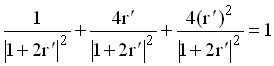# Conservation of Energy for Ohms/square.

Marietta Scientific, Inc.

## Reflection Coefficient

The reflection coefficient G for a thin sheet of ohms/sq. at normal incidences is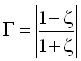where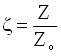and the characteristic impedance of free space is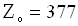The characteristic impedance for a thin sheet is r in parallel with free space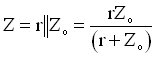and r = resistance (ohms/sq.)

Thus the reflection coefficient is:with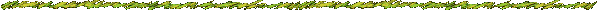## Loss Coefficient

The loss coefficient A is the ratio of the power absorbed in the ohms /sq. to the incident power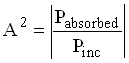where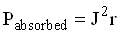andThe physical optics current J for ohms/sq. at normal incidence is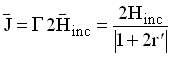where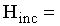incident magnetic field and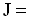sheet current (amps/m)

The power absorbed by the ohms/sq. is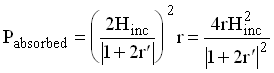Thus the loss coefficient is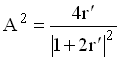or## Transmission Coefficient

The transmission coefficient T is the ratio of the power transmitted to the power incidentwhere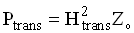The transmitted and scattering fields are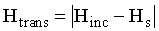and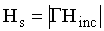thus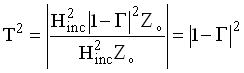or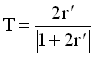## From Conservation of energy

Energy is conserved when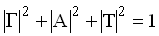Substituting from the above equations